# Implement forward&backward pass from scratch

Mar 01, 2020 · 8 mins read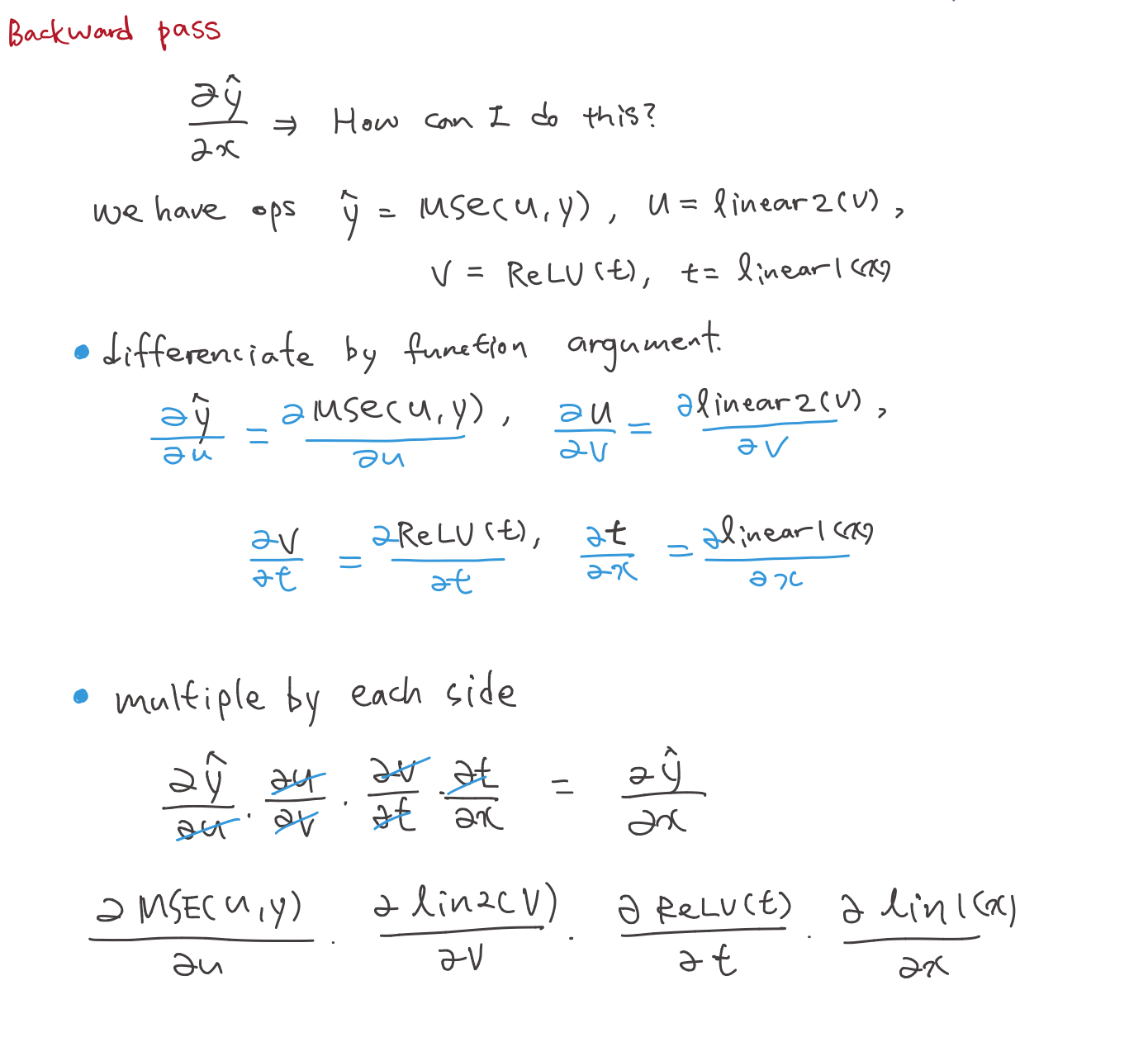Share this

This note is divided into 4 section.

### 1. The forward and backward passes

#### 1.1 Normalization

train_mean,train_std = x_train.mean(),x_train.std()
>>> train_mean,train_std
(tensor(0.1304), tensor(0.3073))


Remember!

• Dataset, which is x_train, mean and standard deviation is not 0&1. But we need them to be which means we should substract means and divide data by std.
• You should not standarlize validation set because training set and validation set should be aparted.
• after normalize, mean is close to zero, and standard deviation is close to 1.

#### 1.2 Variable definition

• n,m: size of the training set
• c: the number of activations we need in our model

### 2. Foundation Version

#### 2.1 Basic architecture

• Our model has one hidden layer, output to have 10 activations, used in cross entropy.
• But in process of building architecture, we will use mean square error, output to have 1 activations and lator change it to cross entropy

• number of hidden unit; 50

see below pic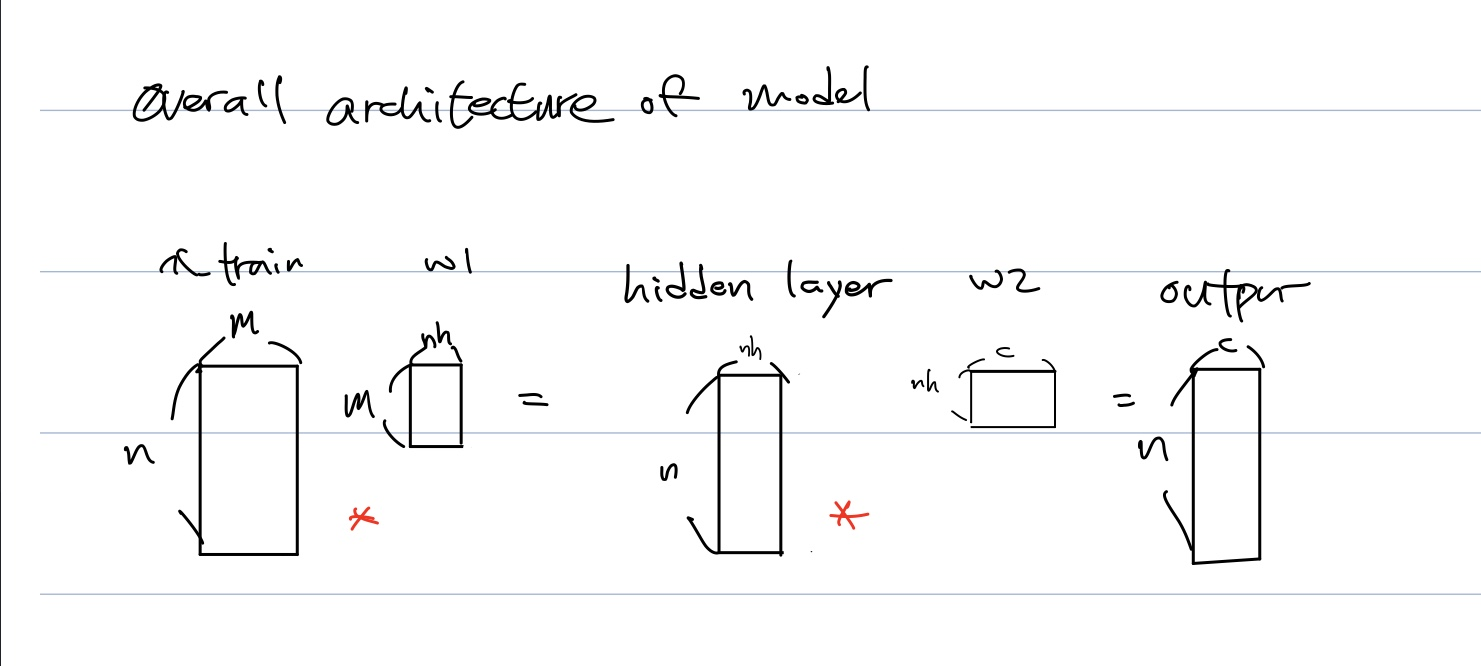• We want to make w1&w2 mean and std be 0&1.
• why initializating and make mean zero and std one is important?
• paper highlighting importance of normalisation - training 10,000 layer network without regularisation1
##### 2.1.1 simplified kaiming init

Q: Why we did init, normalize with only validation data? Because we can not handle and get statistics from each value of x_valid?{: style=”color:red; font-size: 130%; text-align: center;”}

• what about hidden(first) layer?
w1 = torch.randn(m,nh)
b1 = torch.zeros(nh)
t = lin(x_valid, w1, b1) # hidden

>>> t.mean(), t.std()

((tensor(2.3191), tensor(27.0303))


In output(second) layer,

w2 = torch.randn(nh,1)
b2 = torch.zeros(1)
t2 = lin(t, w2, b2) # output

>>> t2.mean(), t2.std()

(tensor(-58.2665), tensor(170.9717))

• which is terribly far from normalzed value.

• But if we apply simplified kaiming init

w1 = torch.randn(m,nh)/math.sqrt(m); b1 = torch.zeros(nh)
w2 = torch.randn(nh,1)/math.sqrt(nh); b2 = torch.zeros(1)
t = lin(x_valid, w1, b1)
t.mean(),t.std()
>>> (tensor(-0.0516), tensor(0.9354))

• But, actually, we use activations not only linear function
• After applying activations relu at linear layer, mean and deviation became 0.5.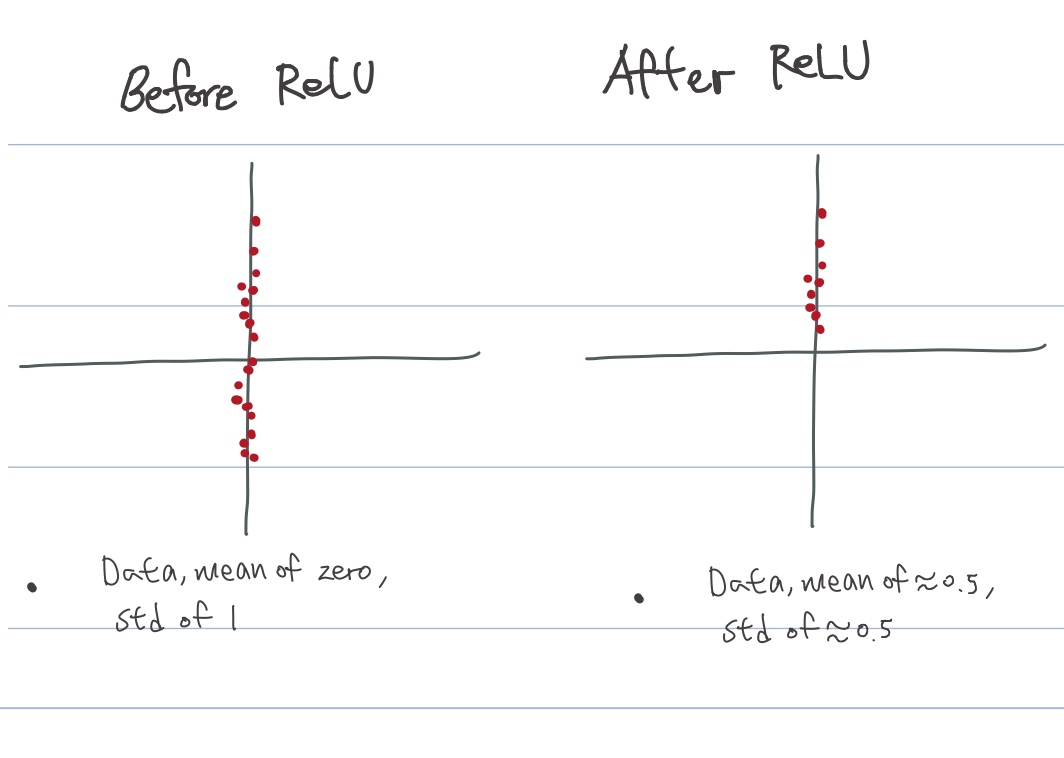##### 2.1.2 Glorrot initialization
• Gaussian(, bell shaped, normal distributions) is not trained very well.
• How to initialize neural nets?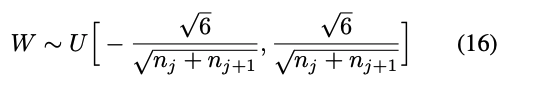with $$n_i$$ the size of layer $$n$$, the number of filters $$i$$.

• But there is No acount for import of ReLU
• If we got 1000 layers, vanishing gradients problem emerges
##### 2.1.3 Kaiming initializating
• Kaiming He, explained here
• rectifier: rectified linear unit
• rectifier network: neural network with rectifier linear units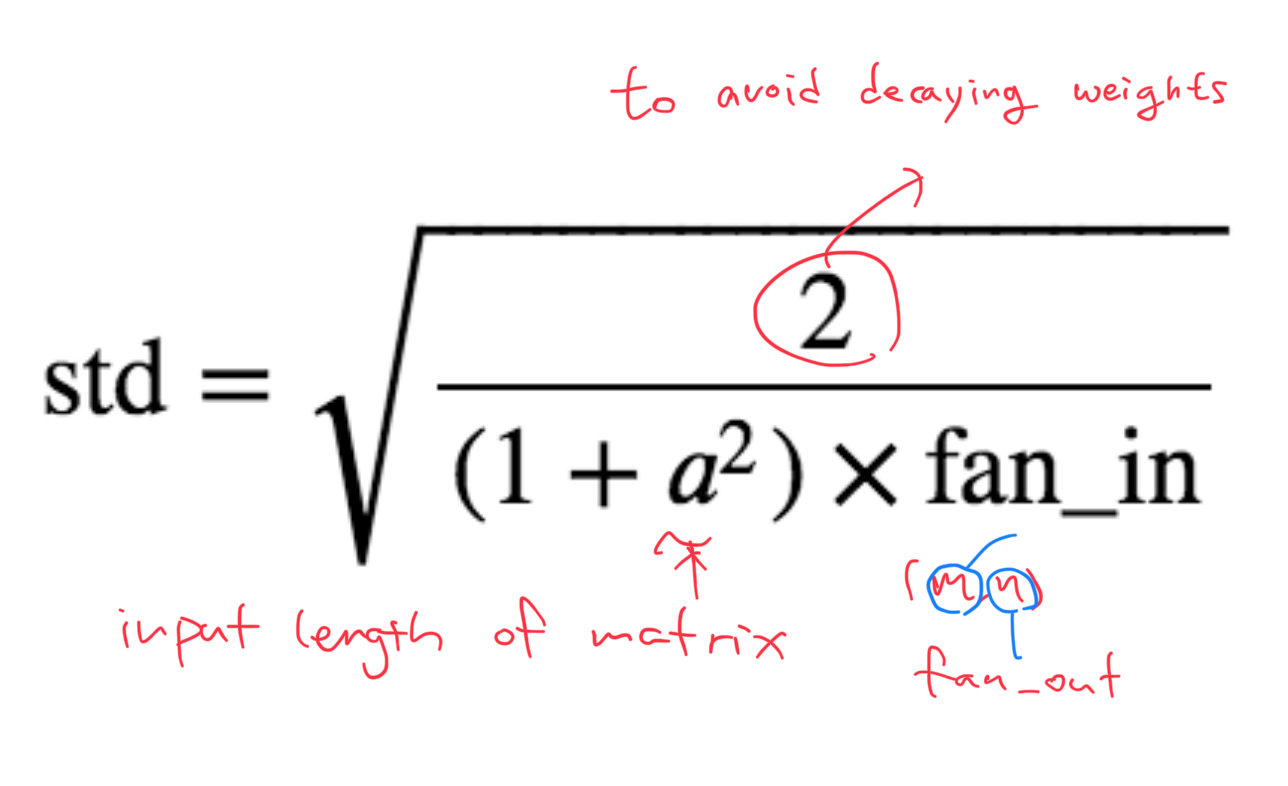• This is kaiming init, and why suddenly replace one to two on a top?
• to avoid vanishing gradient(weights)
• But it doesn’t give very nice mean tough.
##### 2.1.4 Pytorch package
• Why fan_out?
• according to pytorch documentation,

choosing 'fan_in' preserves the magnitude of the variance of the wights in the forward pass.

choosing 'fan_out' preserves the magnitues in the backward pass(, which means matmul; with transposed matrix)

➡️ in the other words, torch use fan_out cz pytorch transpose in linear transformaton.

• What about CNN in Pytorch?

I tried

torch.nn.Conv2d.conv2d_forward??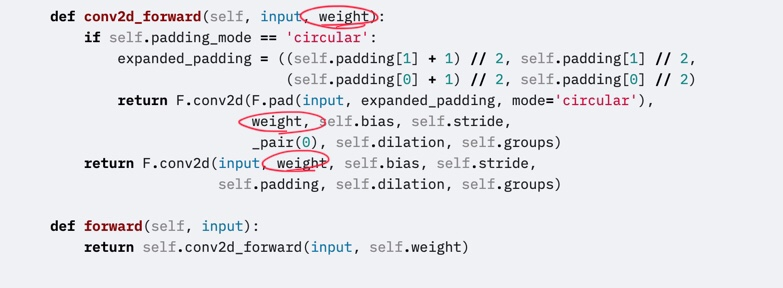Jeremy digged into using

torch.nn.modules.conv._ConvNd.reset_parameters??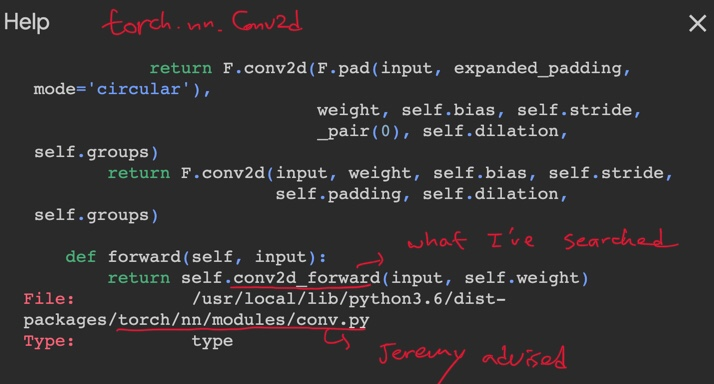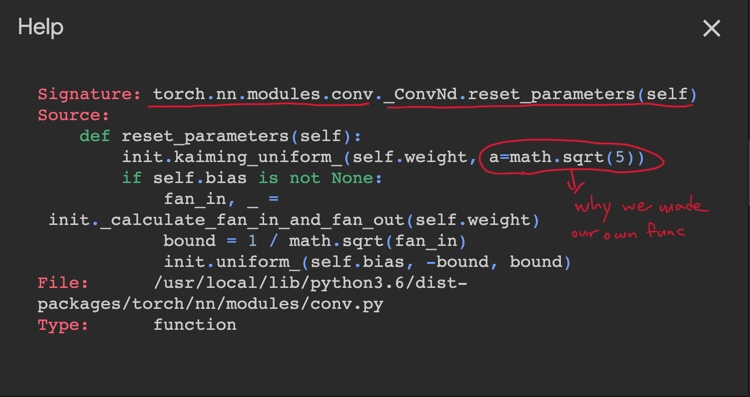2

• in Pytorch, it doesn’t seem to be implemented kaiming init in right formula. so we should use our own operation.
• But actually, this has been discussed in Pytorch community before.3 4
• Jeremy said it enhanced variance also, so I sampled 100 times and counted better results.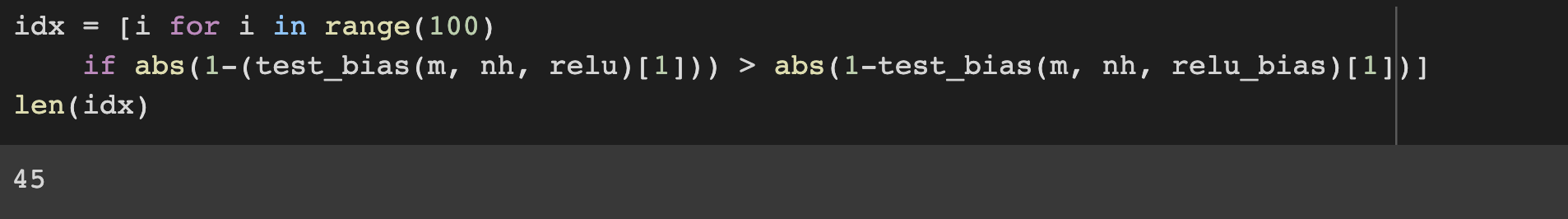• To make sure the shape seems sensible. check with assert. (remember we will replace 1 to 10 in cross entropy)
assert model(x_valid).shape==torch.Size([x_valid.shape,1])
>>> model(x_valid).shape
(10000, 1)

• We have made Relu, init, linear, it seems we can forward pass
• code we need for basic architecture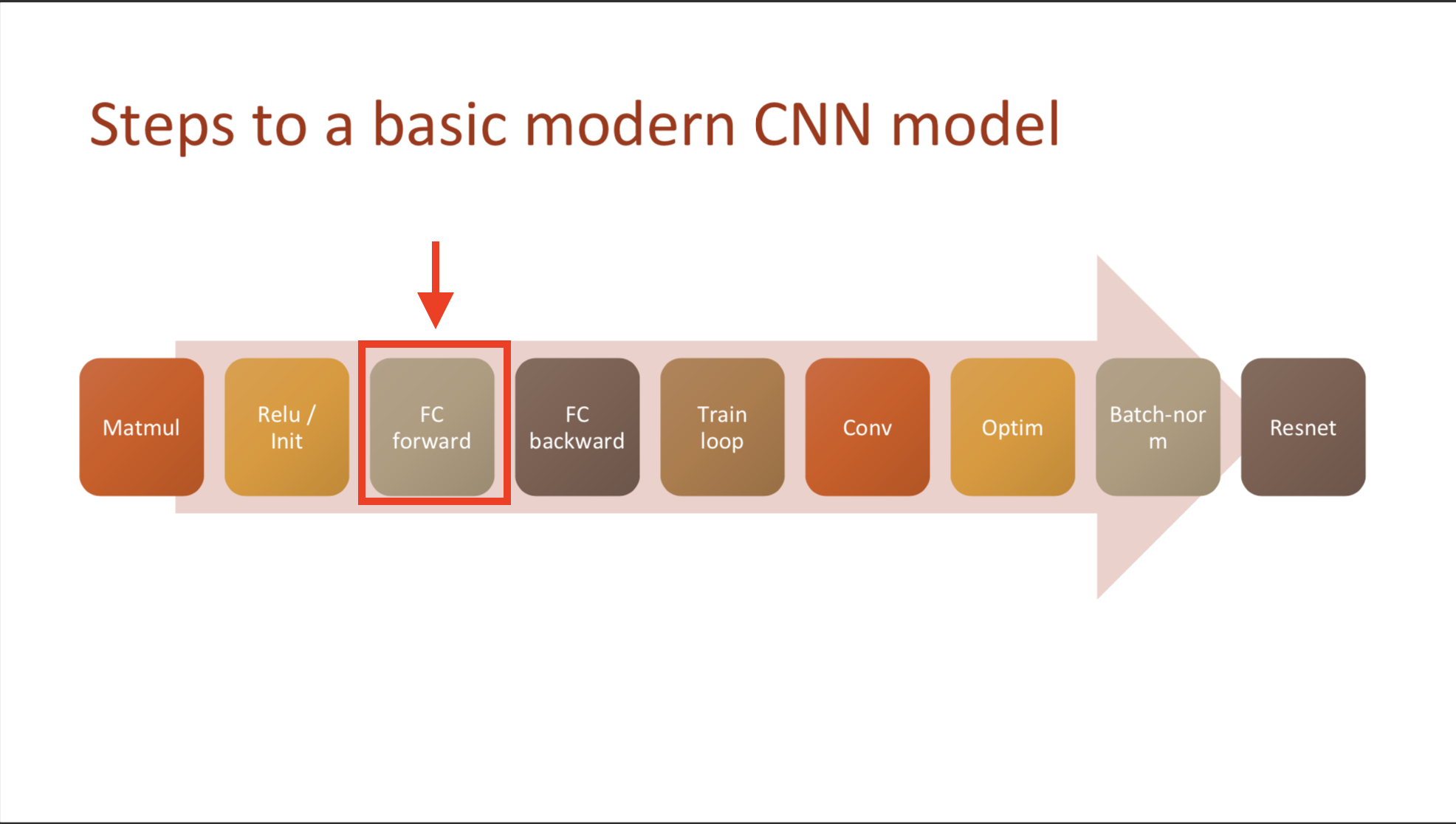nh = 50
def lin(x, w, b): return x@w + b;
w1 = torch.randn(m,nh)*math.sqrt(2./m ); b1 = torch.zeros(nh)
w2 = torch.randn(nh,1); b2 = torch.zeros(1)

def relu(x): return x.clamp_min(0.) - 0.5
t1 = relu(lin(x_valid, w1, b1))

def model(xb):
l1 = lin(xb, w1, b1)
l2 = relu(l1)
l3 = lin(l2, w2, b2)
return l3


#### 2.2 Loss function: MSE

• Mean squared error need unit vector, so we remove unit axis.
def mse(output, targ): return (output.squeeze(-1) - targ).pow(2).mean()

• In python, in case you remove axis, you use ‘squeeze’, or add axis use ‘unsqueeze’
• torch.squeeze where code commonly broken. so, when you use squeeze, clarify dimension axis you want to remove
tmp = torch.tensor([1,1])
tmp.squeeze()
>>> tensor([1, 1])

• make sure to make as float when you calculate

But why??? because it is tensor?{: style=”color:red; font-size: 130%;”}

Here’s the error when I don’t transform the data type

---------------------------------------------------------------------------
TypeError                                 Traceback (most recent call last)
<ipython-input-22-ae6009bef8b4> in <module>()
----> 1 y_train = get_data() # call data again
2 mse(preds, y_train)

TypeError: 'map' object is not subscriptable

• This is forward pass